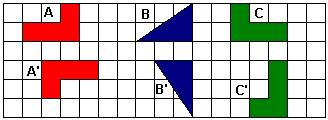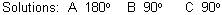# Rotation, Geometry Math Review and Tutorial

math tutorials > rotational geometry math review

## What is rotation? A definition of math rotation

A rotation is the movement of a shape where every point on the shape turns through an angle about a single point called the centre of rotation.

## ExampleBy  convention, the angle of rotation is measured in an anticlockwise direction.  For example, 60 degrees means a rotation 60 degrees anticlockwise, -40 degrees means a rotation of 40 degrees clockwise.

## Geometry problems for you to try.

State the positive angle of rotation of the following: